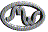# Module `bit32`

Bitwise Operations.

This library provides bitwise operations. It provides all its functions inside the table `bit32`. Unless otherwise stated, all functions accept numeric arguments in the range `(-2^51,+2^51);` each argument is normalized to the remainder of its division by `2^32` and truncated to an integer (in some unspecified way), so that its final value falls in the range `[0,2^32 - 1]`. Similarly, all results are in the range `[0,2^32 - 1]`. Note that `bit32.bnot(0)` is `0xFFFFFFFF`, which is different from `-1`.

## Type `bit32`

 bit32.arshift(x, disp) Returns the number `x` shifted `disp` bits to the right. bit32.band(...) Returns the bitwise `and` of its operands. bit32.bnot(x) Returns the bitwise negation of `x`. bit32.bor(...) Returns the bitwise `or` of its operands. bit32.btest() Returns a boolean signaling whether the bitwise `and` of its operands is different from zero. bit32.bxor(...) Returns the bitwise exclusive `or` of its operands. bit32.extract(n, field, width) Returns the unsigned number formed by the bits `field` to `field + width - 1` from `n`. bit32.lrotate(x, disp) Returns the number `x` rotated `disp` bits to the left. bit32.lshift(x, disp) Returns the number `x` shifted `disp` bits to the left. bit32.replace(n, v, field, width) Returns a copy of `n` with the bits `field` to `field + width - 1` replaced by the value `v`. bit32.rrotate(x, disp) Returns the number `x` rotated `disp` bits to the right. bit32.rshift(x, disp) Returns the number `x` shifted `disp` bits to the right.

## Type `bit32`

### Field(s)

bit32.arshift(x, disp)

Returns the number `x` shifted `disp` bits to the right.

The number `disp` may be any representable integer. Negative displacements shift to the left.

This shift operation is what is called arithmetic shift. Vacant bits on the left are filled with copies of the higher bit of `x`; vacant bits on the right are filled with zeros. In particular, displacements with absolute values higher than 31 result in zero or `0xFFFFFFFF` (all original bits are shifted out).

### Parameters

• `#number x `: value to shift

• `#number disp `: the number of shift, may an positive or negative integer

### Return value

#number: shifted number

bit32.band(...)

Returns the bitwise `and` of its operands.

### Parameter

• `#number ... `: operands

### Return value

#number: bitwise `and` of operands

bit32.bnot(x)

Returns the bitwise negation of `x`.

For any integer `x`, the following identity holds:

``````    assert(bit32.bnot(x) == (-1 - x) % 2^32)

``````

### Parameter

• `#number x `: Number to proceed

### Return value

#number: bitwise negation

bit32.bor(...)

Returns the bitwise `or` of its operands.

### Parameter

• `#number ... `: operands

### Return value

#number: bitwise `or` of operands

bit32.btest()

Returns a boolean signaling whether the bitwise `and` of its operands is different from zero.

### Return value

#boolean: true if the bitwise `and` of its operands is different from zero

bit32.bxor(...)

Returns the bitwise exclusive `or` of its operands.

### Parameter

• `#number ... `: operands

### Return value

#number: bitwise exclusive `or` of its operands.

bit32.extract(n, field, width)

Returns the unsigned number formed by the bits `field` to `field + width - 1` from `n`.

Bits are numbered from `0` (least significant) to `31` (most significant). All accessed bits must be in the range `[0, 31]`.

The default for `width` is `1`.

### Parameters

• `#number n `: input number

• `#number field `: bit field to apply

• `#number width `: the number of bit to take in account (optional, 1 by default)

### Return value

#number: extracted number

bit32.lrotate(x, disp)

Returns the number `x` rotated `disp` bits to the left.

The number `disp` may be any representable integer. For any valid displacement, the following identity holds:

``````assert(bit32.lrotate(x, disp) == bit32.lrotate(x, disp % 32))
``````

In particular, negative displacements rotate to the right.

### Parameters

• `#number x `: original number

• `#number disp `: number of rotate

### Return value

#number: rotated number

bit32.lshift(x, disp)

Returns the number `x` shifted `disp` bits to the left.

The number `disp` may be any representable integer. Negative displacements shift to the right. In any direction, vacant bits are filled with zeros. In particular, displacements with absolute values higher than `31` result in zero (all bits are shifted out). For positive displacements, the following equality holds:

`````` assert(bit32.lshift(b, disp) == (b * 2^disp) % 2^32)
``````

### Parameters

• `#number x `: original number

• `#number disp `: the number of shift

### Return value

#number: shifted number

bit32.replace(n, v, field, width)

Returns a copy of `n` with the bits `field` to `field + width - 1` replaced by the value `v`.

See `bit32.extract` for details about field and width.

### Parameters

• `#number n `: the number to copy

• `#number v `: the value v

• `#number field `: bits field to apply

• `#number width `: the number of bit to take in account (optional, 1 by default)

### Return value

#number: replaced number

bit32.rrotate(x, disp)

Returns the number `x` rotated `disp` bits to the right.

The number `disp` may be any representable integer. For any valid displacement, the following identity holds:

`````` assert(bit32.rrotate(x, disp) == bit32.rrotate(x, disp % 32))
``````

In particular, negative displacements rotate to the left.

### Parameters

• `#number x `: original number

• `#number disp `: number of bits to rotate

### Return value

#number: rotated number

bit32.rshift(x, disp)

Returns the number `x` shifted `disp` bits to the right.

The number `disp` may be any representable integer. Negative displacements shift to the left. In any direction, vacant bits are filled with zeros. In particular, displacements with absolute values higher than `31` result in zero (all bits are shifted out).

For positive displacements, the following equality holds:

`````` assert(bit32.rshift(b, disp) == math.floor(b % 2^32 / 2^disp))
``````

This shift operation is what is called logical shift.

### Parameters

• `#number x `: original number

• `#number disp `: the number of shift

### Return value

#number: shifted number